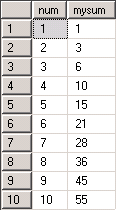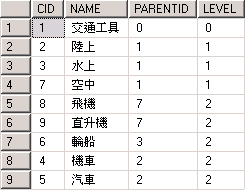## 使用CTE進行遞迴(Recursive)查詢

```WITH cte (num, mysum) AS (
SELECT 1 as num, 1 as mysum
UNION ALL
SELECT num + 1, mysum + num + 1 FROM cte a where num <10
)
SELECT * FROM cte
```是的, 結果就是那個 1 + 2 + 3 + … + 10 = 55 .

```CREATE TABLE CATEGORY (CID int primary key, NAME nvarchar(200), PARENTID int)
INSERT INTO CATEGORY values (1, N'交通工具', 0), (2, N'陸上', 1), (3, N'水上', 1)
INSERT INTO CATEGORY values (4, N'機車', 2), (5, N'汽車', 2), (6, N'輪船', 3)
INSERT INTO CATEGORY values (7, N'空中', 1), (8, N'飛機', 7), (9, N'直升機', 7)
```

```WITH CTE (CID, NAME, PARENTID, LEVEL) AS (
SELECT CID, NAME, PARENTID, 0 FROM CATEGORY WHERE PARENTID = 0
UNION ALL
SELECT A.CID, A.NAME, A.PARENTID, LEVEL + 1 FROM CATEGORY A INNER JOIN CTE B ON A.PARENTID = B.CID
)
SELECT * FROM CTE``````WITH CTE (CID, NAME, PARENTID, LEVEL, ORICID, DATA) AS (
SELECT CID, NAME, PARENTID, 0, CID, CONVERT(NVARCHAR(MAX), NAME) FROM CATEGORY WHERE PARENTID = 0
UNION ALL
SELECT A.CID, A.NAME, A.PARENTID, LEVEL + 1, A.CID, DATA + '-' + A.NAME FROM CATEGORY A INNER JOIN CTE B ON A.PARENTID = B.CID
)
SELECT * FROM CTE
```Types don’t match between the anchor and the recursive part in column “xx” of recursive query “xx”.

## 利用CTE將分頁資料取出-Stored Procedure篇

```create proc sp_getRecordByCTE
@sqlcmd nvarchar(4000),
@ordercmd nvarchar(1000),
@startindex int,
@endindex int
as
declare @mycmd nvarchar(4000)
select @mycmd = '
with myctequery as (
select row_number() over ( ' + @ordercmd + ' )
as CTESN, '+replace(@sqlcmd,'select ',' ')+' )
select * from myctequery
where CTESN between ' + convert(nvarchar,@startindex) + ' and '+convert(nvarchar,@endindex)
exec sp_executesql @mycmd
```

`select a.orderid, a.orderdate, b.custname, b.custaddr from orders a inner join cust b on a.ordercustid = b.custid order by a.orderdate desc`

```exec sp_getRecordByCTE
'select a.orderid, a.orderdate, b.custname, b.custaddr from orders a inner join cust b on a.ordercustid = b.custid',
'order by a.orderdate desc',
51,100```

## 用CTE來取出指定筆數 – SQL2005

SQL2005中有個CTE (Common Table Expression)功能十分強大, 可以用來做遞迴式的參考, 藉以達成複雜的查詢邏輯並簡化查詢指令, 是 SQL2005的一個新的重要功能.